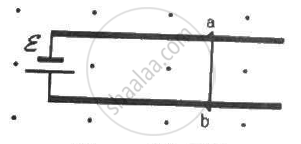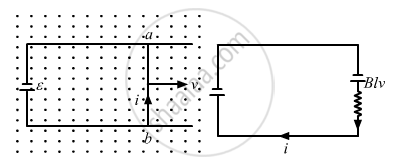Department of Pre-University Education, KarnatakaPUC Karnataka Science Class 12
Advertisement Remove all ads

# Figure Shows a Smooth Pair of Thick Metallic Rails Connected Across a Battery of Emf εHaving a Negligible Internal Resistance. a Wire Ab Of Length L And Resistance R Can Slide Smoothly on the Rails. - Physics

Sum

Figure shows a smooth pair of thick metallic rails connected across a battery of emf εhaving a negligible internal resistance. A wire ab of length l and resistance r can slide smoothly on the rails. The entire system lies in a horizontal plane and is immersed in a uniform vertical magnetic field B. At an instant t, the wire is given a small velocity vtowards right. (a) Find the current in it at this instant. What is the direction of the current? (b) What is the force acting on the wire at this instant? (c) Show that after some time the wire ab will slide with a constant velocity. Find this velocity.Advertisement Remove all ads

#### Solution

According to Fleming's left hand rule the force in the wire ab will be in the upward direction.

Moreover, a moving wire ab is equivalent to a battery of emf vBl as shown in the figure.At the given instant, the net emf across the wire (e) is E − Bvl.

(a) The current through the wire is given by

$i = \frac{E - Bvl}{r}$

The direction of the current is from b to a.

(b) The force acting on the wire at the given instant is given by

$F = ilB = \left( \frac{E - Bvl}{r} \right)$towards right

(c) The velocity of the wire attains a value such that it satisfies E = Bvl.

The net force on the wire becomes zero. Thus, the wire moves with a constant velocity v.

$\therefore v = \frac{E}{Bl}$

Concept: Motion in Combined Electric and Magnetic Fields - Velocity Selector
Is there an error in this question or solution?
Advertisement Remove all ads

#### APPEARS IN

HC Verma Class 11, Class 12 Concepts of Physics Vol. 2
Chapter 16 Electromagnetic Induction
Q 49 | Page 310
Advertisement Remove all ads
Advertisement Remove all ads
Share
Notifications

View all notifications

Forgot password?